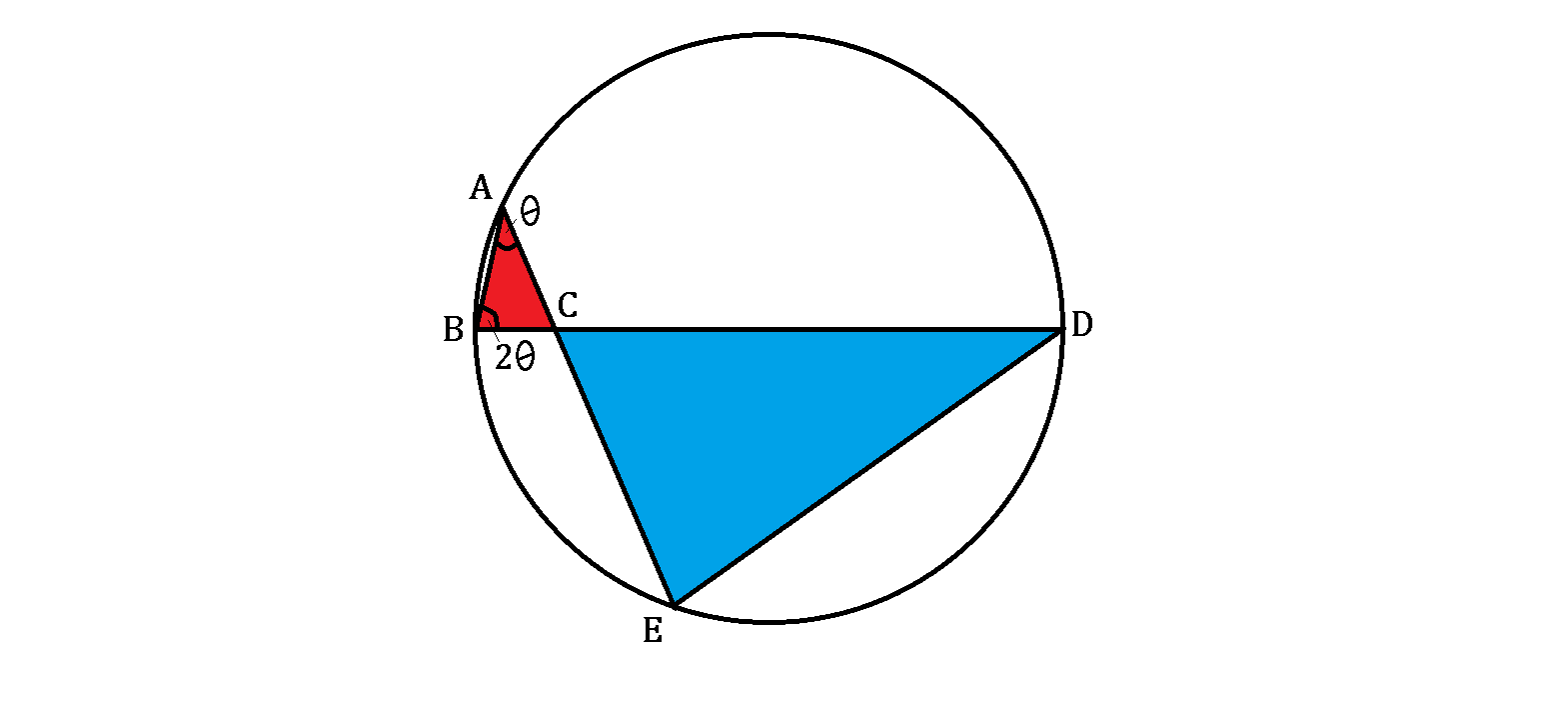# Butterfly EffectThe lines above are drawn joining points $A, B, D, E$ on the circumference of the circle, such that $BD$ is a diameter of length $20$ and $\angle ABC = 2\angle BAC.$

If $AC = 3$, what's the ratio of the areas of the two triangles, blue $\triangle CDE$ to red $\triangle ABC?$

×